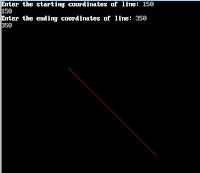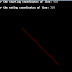Line Drawing using DDA Algorithm with patterns such as simple,dash,dash-dot,thick
Algorithm for DDA(Digital Differential Algorithm)

1. Read the lines and points (x1,y1) and (x2,y2) such that they are not equal.(If they are equal then plot those points and exit)
2. D(x)= | x2-x1 and | D( y)=| y2-y1 |
3. Find out the length (if D(x) >= D(y), then length equal to D(x)).
4. D(x)=(x2-x1/length), D(x)=(x2-x1/length)
5. x=x1+0.5(sign(D(x))) y=y1+0.5(sign(D(y))
6. (Here sign function make algorithm work in all quadrants, if it returns -1, 0, 1 depending on whether its argument is less than zero, equal to zero or greater than zero)
7. Plot int x,int y;
8. i=1;
9. while(i<=length)
10. x=x+D(x); y=y+D(y);
11. plot int x,int y;
12. i++;
13. }
14. STOP.
```//program for DDA

#include <stdio .h="">
#include <dos .h="">
#include <graphics .h="">

void lineDDA(int, int, int, int);

void main()
{
int x1, y1, xn, yn;
int gd = DETECT, gm;
initgraph(&gd, &gm, "c:\\tc\\bgi");
printf("Enter the starting coordinates of line: ");
scanf("%d %d", &x1, &y1);
printf("Enter the ending coordinates of line: ");
scanf("%d %d", &xn, &yn);
lineDDA(x1, y1, xn, yn);
getch();
}

void lineDDA(int x1, int y1, int xn, int yn)
{
int dx, dy, m, i;
m = (yn-y1)/(xn-x1);
for (i=x1; i<=xn; i++)
{
if (m <= 1)
{
dx = 1;
dy = m * dx;
}
else
{
dy = 1;
dx = dy / m;
}
x1 = x1 + dx;
y1 = y1 + dy;
putpixel(x1, y1, RED);
delay(20);
}
}
</graphics></dos></stdio>```
Output:CG - 1.1- Line Drawing using DDA AlgorithmReviewed by Huzaif Sayyed on August 18, 2017 Rating: 5